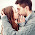# How to convert Amount in Words using Crystal Report?

How to Convert Amount (Currency) in words directly on Crystal Report.

Just Simply copy paste below code in your function.
To Create function follow the steps given below:
i) go to Field Explorer
ii) right click on Formula Fields click on New give specific name
iii) Formula Editor window will open just paste the Code given below
iv) Save & Close...
v) Just drag the Field to your specific required Location that done...

i) vwCostTypes.UnitCost : This is a Database Field. Change as per your requirement.
Please note if you have Currency as Datatype in SQL DB than Do use ToNumber() function to convert it to number otherwise it will throw error of Number Required.

//#####################

//Created by Anup Shah.
//#####################
numbervar RmVal:=0;
currencyVar Amt:=0;
numbervar pAmt:=0;
stringvar InWords :="Rupees ";
Amt := ({vwCostTypes.UnitCost});

if Amt > 10000000 then RmVal := truncate(ToNumber(Amt)/10000000);
if Amt = 10000000 then RmVal := 1;
if RmVal = 1 then
InWords := InWords + " " + towords(RmVal,0) + " crore"
else
if RmVal > 1 then InWords := InWords + " " + towords(RmVal,0) + " crores";

Amt := Amt - Rmval * 10000000;
if Amt > 100000 then RmVal := truncate(ToNumber(Amt)/100000);
if Amt = 100000 then RmVal := 1;
if RmVal >=1 then
InWords := InWords + " " + towords(RmVal,0) + " lakhs";

Amt := Amt - Rmval * 100000;
if Amt > 0 then InWords := InWords + " " + towords(truncate(Amt),0);
pAmt := (ToNumber(Amt) - truncate(ToNumber(Amt))) * 100;
if pAmt > 0 then
InWords := InWords + " and " + towords(pAmt,0) + " paisa only"
else
InWords := InWords + " only";
UPPERCASE(InWords)
//#####################

Happy Coding...!!! ;)

1.Thanks For Coding

2."(TOTAL EURO "+UpperCase(Towords(sum({@Tot}),0))+" Point "+ UpperCase(Towords(remainder(sum({@Tot}),1)*100,0))

3.It's work very nice,

Thank you.

4.Nice and Amazing. Thank you

5.Thanks for ya Code but how do u write it to convert into shillings

6.FOr 4 Crores it showing 4 crore 4 lakh only. there is some bug in it

1.Did you get any response for this problem?

7.RUPEES TWO LAKHS THIRTY-FIVE THOUSAND FOUR HUNDRED FOURTEEN ONLY

how to remove - between thirty and five

8.9.Thank you.......

10.Great Thinking and Coding man ....
So simple to use

11.Does not work properly more than 1 crore. please try 50000000.

12.I read that Post and got it fine and informative. convert money calculator

13.Thanks, that was a really cool read! convert currency